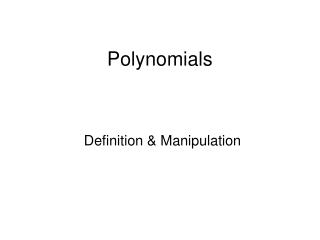DownloadDownload PresentationPolynomials

# Polynomials

Télécharger la présentation## Polynomials

- - - - - - - - - - - - - - - - - - - - - - - - - - - E N D - - - - - - - - - - - - - - - - - - - - - - - - - - -
##### Presentation Transcript

1. Polynomials Definition & Manipulation

2. What is a Polynomial? two x2 + 5x – 2 is a polynomial of degree 2x5+ 5x - 2 is a polynomial of degree five The degree of a polynomial is the index of the highest power of x in the polynomial x3 + 2x2 + x - 4 has degree three 10x9 - x2 + x + 1 has degree nine

3. 3x2 + 5x4 – 2x + 1 5x4 + 3x2 – 2x + 1 The coefficient of a term is the number in front of the term. It is practice to place the terms of a polynomial in order of decreasing indices with the constant term at the end.(The constant is the x0 term). • Note: • No term in a polynomial has an index less than zero. i.e. no negative indices. • A polynomial is an expression in only ONE variable (x).

4. Evaluating a Polynomial What is the value of the polynomial 4x4 +7x2 -2x +1 when x=2 ? i.e. f(x) = 4x4 +7x2 -2x +1 f(2) = ? This can be done(a) by substitution FILL THE DETAILS (b) Using a “multiply and add table”

5. For the polynomial ax4 + bx3 + cx2 + dx +e the table looks like this ????????

6. Dividing a polynomial by (x – h) or (x + h) To do this we use synthetic division. We put the coefficients of the polynomial into the table and “h” outside. In the bottom of the table, the last term is the remainder and the other terms are the coefficients of the quotient. The degree of the quotient is one less than the degree of the polynomial. Example 1: 17 ÷ 3 = 5 r 2 Example 2: Find the quotient and remainder when x3+ 6x2 + 3x -15 ÷ (x + 3) FILL IN

7. Dividing a polynomial by (kx – h) or (kx + h) 1st We rearrange 2nd We use synthetic division to divide by 3rd We divide the quotient by k Example 3: Divide FILL IN !!!

8. The Remainder Theorem The remainder on dividing We have seen that we can find the value of f(x) by using a “multiply and add” table, where the last entry is f(h). We have also seen that this same table can be used for calculating the quotient and remainder when dividing, where the last entry is the remainder. The remainder = f(h)

9. Proof The remainder on dividing If we only need a remainder we can either use “synthetic division” or evaluate f(h). If you need Quotient and Remainder then stick to using “synthetic division” as evaluation of f(h) will only give the remainder.

10. The Factor Theorem • If (x – h) is a factor of f(x) thenthe remainder on dividing f(x) by (x - h) is 0. i.e. f(h) = 0 • (2) If the remainder on division by (x – h) is zero, i.e. f(h)= 0,then (x - h) is a factor. Example(s) !!!!!!!

11. Finding Polynomial Coefficients Examples Galore !!!!!

12. Solving Polynomial Equations A polynomial equation looks like this: We have been solving Polynomial equations of degree 1 and degree 2 for sometime. These are more commonly called Linear and Quadratic equations respectively. When we solve an equation with zero on the right hand side, we are trying to find the roots of the equation in other words where the graph cuts the x-axis.